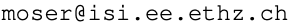# Abstract

A technique is proposed for the derivation of upper bounds on
channel capacity. It is based on a dual expression for channel
capacity where the maximization (of mutual information) over
distributions on the channel input alphabet is replaced with a
minimization (of average relative entropy) over distributions
on the channel output alphabet. We also propose a technique
for the analysis of the asymptotic capacity of cost-constrained
channels. The technique is based on the observation that under
fairly mild conditions capacity achieving input distributions
"escape to infinity."

The above techniques are applied to multiple-antenna flat fading
channels with memory where the realization of the fading process
is unknown at the transmitter and unknown (or only partially known)
at the receiver. It is demonstrated that, for high signal-to-noise
ratio (SNR), the capacity of such channels typically grows only
double-logarithmically in the SNR. To better understand this
phenomenon and the rates at which it occurs, we introduce the fading
number as the second order term in the high-SNR asymptotic expansion
of capacity, and derive estimates on its value for various systems.
It is suggested that at rates that are significantly higher than the
fading number, communication becomes extremely power inefficient,
thus posing a practical limit on practically achievable rates.

Upper and lower bounds on the fading number are also presented. For
single-input single-output (SISO) systems the bounds coincide, thus
yielding a complete characterization of the fading number for general
stationary and ergodic fading processes. We also demonstrate that for
memoryless multiple-input single-output (MISO) channels, the fading
number is achievable using beam-forming, and we derive an expression
for the optimal beam direction. This direction depends on the fading
law and is, in general, not the direction that maximizes the SNR on
the induced SISO channel. Using a new closed-form expression for the
expectation of the logarithm of a noncentral chi-square distributed
random variable we provide some closed-form expressions for the
systems with circularly symmetric stationary and ergodic Gaussian
mean, fading variance, and the mean squared-error in predicting the
present fading from its past; it is not directly related to the

For the Rayleigh, Ricean, and multiple-antenna Rayleigh fading channels
we also present firm (nonasymptotic) upper and lower bounds on
channel capacity. These bounds are asymptotically tight in the sense
that their difference from capacity approaches zero at high SNR, and
their ratio to capacity approaches one at low SNR.

# Keywords

-||-   _|_ _|_     /    __|__   Stefan M. Moser